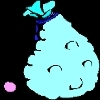You may also likeInequalities

A bag contains 12 marbles. There are more red than green but green and blue together exceed the reds. The total of yellow and green marbles is more than the total of red and blue. How many of each colour there are in the bag?What's it Worth?

There are lots of different methods to find out what the shapes are worth - how many can you find?Turnips

Baldrick could buy 6 parsnips and 7 turnips, or 8 parsnips and 4 turnips. How many parsnips could he buy?

Shape Sums

Age 11 to 14 Short Challenge Level:

$\Box = 2 \bigtriangleup$, from the first equation.
Then, $\bigcirc = \Box + \bigtriangleup = 2\bigtriangleup + \bigtriangleup = 3\bigtriangleup$.
Then, $\Diamond = \bigcirc + \Box + \bigtriangleup = 3\bigtriangleup + 2\bigtriangleup + \bigtriangleup = 6 \bigtriangleup$.
Therefore, $\Diamond$ is worth $6 \bigtriangleup$.